﻿ Research and Mitigation on EMI Noise for PV Converter

### Research and Mitigation on EMI Noise for PV Converter

Li Lin, Yan Wei, Qiu Dongmei

## Research and Mitigation on EMI Noise for PV Converter

Li Lin1, Yan Wei2, 3,, Qiu Dongmei1

1Jiangsu Institute of Metrology, Nanjing, China

2School of Electrical & Automation Engineering, Nanjing Normal University, Nanjing, China

3Key Lab. of Advanced Technology for Small & Medium UAV, Nanjing University of Aeronautics & Astronautics, Nanjing, China

### Abstract

Photovoltaic (PV) systems have been widely used for power generation and renewable energy. Conducted electromagnetic interference (EMI) noise have generated, which affect the performance of other device and the grid. In the paper, the measurement uncertainty of artificial mains network (AMN) has been analyzed. The conducted EMI noise mechanism and noise mitigation methods have been proposed, including transistor filter and well grounding design strategies. The simulation and experiment results show that the conducted EMI noise generated by a certain convert can be suppressed well and pass EN 55011 and EN 55022 standards, thus good realization and validation.

• Li Lin, Yan Wei, Qiu Dongmei. Research and Mitigation on EMI Noise for PV Converter. American Journal of Electrical and Electronic Engineering. Vol. 5, No. 2, 2017, pp 34-39. http://pubs.sciepub.com/ajeee/5/2/1
• Lin, Li, Yan Wei, and Qiu Dongmei. "Research and Mitigation on EMI Noise for PV Converter." American Journal of Electrical and Electronic Engineering 5.2 (2017): 34-39.
• Lin, L. , Wei, Y. , & Dongmei, Q. (2017). Research and Mitigation on EMI Noise for PV Converter. American Journal of Electrical and Electronic Engineering, 5(2), 34-39.
• Lin, Li, Yan Wei, and Qiu Dongmei. "Research and Mitigation on EMI Noise for PV Converter." American Journal of Electrical and Electronic Engineering 5, no. 2 (2017): 34-39.

 Import into BibTeX Import into EndNote Import into RefMan Import into RefWorks

123
Prev Next

### 1. Introduction

For energy safety and renewable, photovoltaic systems have been widely used for power generation [1, 2, 3]. The direct current (DC) can be obtained from photo through solar cells. And the DC signals transfer to the alternating current (AC) signals by employing the photovoltaic converters. Due to the performance and operation of PV converters, much conducted EMI noises are generated based on switch mode transistors, control strategy, high frequency chips, parasitic inductance and capacitors and topology [4, 5, 6, 7, 8], as shown in Figure 1.Download asVeiw figureFigures index
Figure 1. The topology of PV converter

Firstly, the transistors T11, T12, T21, T22, T31, T32 can generate very shape and steep current pulse di/dt through switch-on and switch-off motion. And the pulse can couple into the output current and the grid. In general, the spectrum of the pulse is from several kilo hertz to several mega hertz. The time domain and frequency domain pulse wave is decided by the control strategy, including wave pattern, width, duty ratio and period.

Secondly, the conducted EMI noises above several mega hertz are produced by the high frequency chips, such as crystal oscillator, single chip microcomputer and signal acquisition chips.

Thirdly, the envelope of conducted EMI noises increases totally due to the grounding modes, including single point grounding, multi points grounding and hybrid grounding.

The last but not the least, the conducted EMI noises are generated by the topology of PV converter, such as circuit loop, parallel routing and so on.

The conducted EMI noise mechanism are coupled and complicated due to the above four complicated reasons. Many researches have been proposed to study and solve the above problems. An AC EMI filter structure in the single-phase grid-interface converter was analyzed for low-voltage dc distribution system, which helps reduce the coupling between dc and ac-side, and thus simplifies the CM EMI filter design process . A new inverter topology, buck-buck-boost (B3) inverter, which is derived from an H-bridge converter with additional switch legs, was designed to reduce the common mode noise due to its solid connection between the grounds of the input and output terminals . A digital active EMI filter (DAEF), whose control technique is concurrently implemented with a digital controller of a grid-tied photovoltaic microinverter, was proposed to suppress common mode EMI noises. And the DAEF can achieve an equivalent or better performance in terms of EMI suppression and maintain stability within the operation bandwidth . The performance of spread spectrum frequency modulation (SSFM) was evaluated to the EMI reduction of a real power converter that uses periodic pattern switching frequency modulation . A new method is proposed to test the conducted and radiated electromagnetic interference (EMI) noise of photovoltaic invert based on analysis the internal structure and measuring principle of artificial mains network .

To solve the conducted EMI noise problems of PV converter, the measurement method, noise mechanism and noise mitigation approaches are analyzed and proposed in the paper.

### 2. Measurement Uncertainty Based on Artificial Mains Network

According to EN 55011 and EN 55022, conducted EMI noises should be determined by EMI receiver and artificial mains network (AMN), where both quasi peak detector and average detector are fixed on the EMI receiver. The setup of conducted EMI noise measurement for single phase was shown in Figure 2.Download asVeiw figureFigures index
Figure 2. Conducted EMI noise measurement under EN 55011/ EN 55022

Due to the parasitic capacitor and inductance of AMN, the test result of conducted EMI noise was uncertainty. According to CISPR 16, the topology structure is shown in Figure 3(a), where C1 is 1μF, C2 is 0.1μF, R1 is 50Ω, R2 is 1kΩ and L1 is 50μH. Concerned on the parasitic parameters of the above capacitors, inductances and resistances, the equivalent HF circuit was shown in Figure 3(b), where Lesc1 and Lesc2 are the parasitic inductance of C1 and C2, respectively. Cesl1 is the parasitic capacitor of L1. Les and Res are the parasitic inductance and resistance of the interface adapter.Download asVeiw figureFigures index
Figure 3. The topology and parasitic parameters of AMN

As shown in Figure 3, any parasitic parameters can be ignored due to the design and manufacturing technique of AMN, but the parasitic inductance of the interface adapter Les should be concerned for the different interfaces and converters.

To determine the impedance under PV converter side of AMN, Les was considered as 10nH and 100nH, respectively, and the other parasitic parameters can be neglected due to the measurement circuit, as shown in Figure 4. The simulation results show that with the Les and measurement frequency increasing, the impedance under SMPS side was increasing greatly.

Therefore, the proper AMN and the interface adapters should be designed and employed to determine the conducted EMI noise and decrease the uncertainty of the measurement.Download asVeiw figureFigures index
Figure 4. The impedance under PV converter side of AMN concerned on the parasitic parameters

### 3. Noise Mechanism Identification

According to the different noise transmission paths, the conducted EMI noises can be considered as common mode (CM) noise and differential mode (DM) noises, where the CM noise is between live/neutral and ground lines, and the DM noise is between live and neutral lines.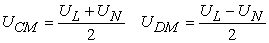(1)

where, UCM and UDM represent CM and DM noises, UL and UN denote live and neutral noises, respectively.

To reach the Eq(1) algorithm, four style of noise separation networks (NSN) were designed by Paul, See, Mardiguian and Guo based on high frequency transformer or power combiner/divider. To improve its characteristic and performance, a novel topology of NSN has been proposed, as shown in Figure 5. The voltage at the primary side of the high frequency transformer is the sum of the UL and UN. And the voltage at the secondary side of the high frequency transformer is the difference between UL and UN, which is consistent with the formula (1). Moreover, the CM choke at the secondary side can be mitigate the CM current, which will improve the NSN performance. The NSN can be placed between AMN and EMI receiver to extract the CM and DM noise.Download asVeiw figureFigures index
Figure 5. A novel topology of NSN

To compare with the proposed NSN, Paul NSN, See NSN, Mardiguian NSN and GUO NSN, the performance characteristic of the NSN should be measured. By employing the vector network analyzer (VNA), the CM insertion loss (CMIL), DM insertion loss (DMIL), CM rejection ratio (CMRR) and DM rejection ratio (DMRR) have been obtained, as shown in Table 1. The CMIL, DMIL, CMRR and DMRR is better than other type NSNs.

#### Table 1. Characteristic and performance of the different NSNsDownload asPowerPoint Slide

Veiw figure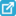View current table in a new window

The novel NSN has been used to extract the CM and DM noises generated from switched reluctance motor (SRM), whose rated voltage and power are 220V and 3kW, respectively. But the loads of the SRM are 1kW and 2.5kW. As shown in Figure 6, the experiment results demonstrate the novel NSN has good performance and validation.Download asVeiw figureFigures index
Figure 6. The topology and parasitic parameters of AMN

### 4. Noise Reduction Scheme

The different noise reduction methods should be used to solve the CM and DM noises, such as CM and DM filters. Two situations have been analyzed as follows.

4.1. Transistor Filtering

The switch velocity and frequency are controlled under different strategies, while some high frequency noises from 9kHz to 30MHz can be coupled into the power line. To reduce the high frequency noises, the filter capacitor can be shunt between the collector and emitting electrodes the bipolar junction transistor (BJT), similarly, the filter capacitor can also be shunt between the source and drain electrodes of the metal oxide semiconductor field effect transistor (MOSFET).

Moreover, the value of the capacitor should be smaller than 0.1μF not only for the noise mitigation but also for the safety regulations & design.

4.2. Well Grounding

No well grounding can generate much more conducted noises, especially the CM noises, whose equivalent transmission circuit is shown in Figure 7(a). The measurement result of the load can be derived from Eq.(2).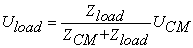(2)

where, UCM denotes the equivalent voltage source of CM noise, Uload represents the measurement result of the load, ZCM and Zload signify the equivalent impedance of the CM noise and the load. As shown in Figure 7(b), when the load was floating ground, the capacitor between floating ground and ground should be considered. And the measurement result of the equivalent load can be derived from Eq.(3).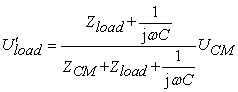(3)

where, U’load represents the measurement result of the equivalent load, C denotes the parasitic capacitor between floating ground and ground.

As shown in Figure 7 and Eq.(2-3), the CM noise generated from no well grounding can be obtained.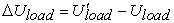(4)

where, ∆Uload expresses the CM noise generated from no well grounding.Download asVeiw figureFigures index
Figure 7. CM noise generated from no well grounding

To solve the above problem, the PCB should be well grounding, especially the DC power supply, such as 5V, 3.3V, 1.8V, 1.2V and so on. In practice, some ferrite beads were used to protect the mutual interference between the above DC power supplies, as shown in Figure 8(a), where U1=U2, C1=C2=22μF, C3=C4=C5=C6=0.1μF.Download asVeiw figureFigures index
Figure 8. CM noise mitigation method for no well grounding

The high frequency impedance should be considered due to the two ferrite beads. To solve the problems, the two ferrite beads were removed and two capacitors were placed, as shown in Figure 8(b), where C7=100pF, C8=10pF. And the high frequency impedance between DC power supplies can be ignored.

### 5. Experiment & Verification

To verify the proposed methods, the conducted EMI noise generated from the converter is analyzed in the paper. According to the EN 55022, R&S EMI receiver ESL3 and R&S artificial mains network (AMN) ENV216 are used to measure the conducted EMI noise.

The original conducted EMI noise of the converter is shown in Figure 9(a), and the noise can’t pass EN 55022 class B. The Quasi peak (QP) and average value (AV) of the conducted EMI noises are very high. To solve the conducted EMI noise problem, the mitigation approaches are proposed as follows:

1) EMI filter for the converter is designed, as shown in Figure 9(b), where L1 denotes 10 mH CM choke, both L2 and L3 denote 1mH inductance, C1, C2, C3 and C4 signify 0.033μF, 0.01nF ,10nF and 1nF, respectively.

2) 10nF capacitor is shunt between the source and drain electrodes of the MOSFET.

3) The ground plane is designed for PCB to well grounding.

As shown in Figure 9(c), by employing the above mitigation method, the conducted EMI noise of the converter was decreased significantly and can pass the EN 55022 Class B, which verify the present method effectiveness and validation.Download asVeiw figureFigures index
Figure 9. Conducted EMI noise experiment and verification for converter

### 6. Conclusion

In the paper, the measurement uncertainty, noise mechanism and mitigation method for conducted EMI noise are proposed to solve the EMI problem for the PV converter.

1) The uncertainty of the AMN under PV converter side is analyzed due to high frequency parasitic inductance of the interface adapter, which can improve measurement accuracy.

2) A novel NSN is designed to identify the CM and DM noises from the total conducted EMI noises, which can obtain the noise mechanism and its reduction methods.

3) Transistor filtering and well ground methods are proposed to analyze and mitigate the conducted EMI noise generated from the PV converters.

4) The mitigation methods have been used to a certain convert, which can suppress the conducted EMI noise well and pass the EN 55022 Class B standard.

### Acknowledgements

The paper is supported by National Science Foundation of Jiangsu Province (BK20161019).

### References

  Sugihara H., Yokoyama K., Saeki O., et al, “Economic and Efficient Voltage Management Using Customer-Owned Energy Storage Systems in a Distribution Network With High Penetration of Photovoltaic Systems”, Power Systems, IEEE Transactions on, 28 (1), 102-111, 2013.In article View Article  Kai Sun, Li Zhang, Yan Xing, et al, “A Distributed Control Strategy Based on DC Bus Signaling for Modular Photovoltaic Generation Systems With Battery Energy Storage”, Power Electronics, IEEE Transactions on, 26 (10). 3032-3045, 2011.In article View Article  Jabr R.A., “Minimum loss operation of distribution networks with photovoltaic generation”, Renewable Power Generation, IET, 8 (1). 33-44, 2014.In article View Article  Chao K.H., Huang C.H., “Bidirectional DC-DC soft-switching converter for stand-alone photovoltaic power generation systems”, Power Electronics, IET, 7 (6). 1557-1565, 2014.In article View Article  Gu B., Dominic J., Lai J.S., et al, “High Boost Ratio Hybrid Transformer DC–DC Converter for Photovoltaic Module Applications”, Power Electronics, IEEE Transactions on, 28 (4). 2048-2058., 2013.In article View Article  Leppaaho J., Suntio T., “Characterizing the Dynamics of the Peak-Current-Mode-Controlled Buck-Power-Stage Converter in Photovoltaic Applications”, Power Electronics, IEEE Transactions on, 29 (7). 3840-3847, 2014.In article View Article  Kuo-Ching Tseng, Chi-Chih Huang, Wei-Yuan Shih, “A High Step-Up Converter With a Voltage Multiplier Module for a Photovoltaic System”, Power Electronics, IEEE Transactions on, 28 (6). 3047-3057, 2013.In article View Article  Jong-Pil Lee, Byung-Duk Min, Tae-Jin Kim, et al, “A Novel Topology for Photovoltaic DC/DC Full-Bridge Converter With Flat Efficiency Under Wide PV Module Voltage and Load Range”, Industrial Electronics, IEEE Transactions on, 55 (7). 2655-2663, 2008.In article View Article  Dong Dong, Xuning Zhang, Fang Luo, et al, “Common-mode EMI noise reduction for grid-interface converter in low-voltage DC distribution system”, Applied Power Electronics Conference and Exposition (APEC), 2012 Twenty-Seventh Annual IEEE, Orlando, FL, United states, 451-457, 2012.In article PubMed  Shin J.W., Shin H., Seo G.S., et al, “Low-Common Mode Voltage H-Bridge Converter with Additional Switch Legs”, Power Electronics, IEEE Transactions on, 28 (4). 1773-1782, 2013.In article View Article  Hamza D., Mei Qiu, Jain P.K., “Application and Stability Analysis of a Novel Digital Active EMI Filter Used in a Grid-Tied PV Microinverter Module”, Power Electronics, IEEE Transactions on, 28 (6). 2867-2874, 2013.In article View Article  Gonzalez D., Bialasiewicz J.T., Balcells J., et al, “Wavelet-Based Performance Evaluation of Power Converters Operating With Modulated Switching Frequency”, Industrial Electronics, IEEE Transactions on, 55 (8). 3167-3176, 2008.In article View Article  Dong Ying Hua, Liu Mei Yin, Cao Lei, “EMI noise testing and diagnosis for PV inverter”, 2013 International Conference on Advances in Energy and Environmental Science, ICAEES 2013, Guangzhou, China, 31-35, 2013.In article View Article